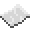# math.max

math.max
Function
Syntax
math.max(
• numbers... : number
)

Returns number
API math
Source Lua (source)

Return the greatest of all the numbers given.ExamplePrint the maximum value of user input
Read as many lines as the user inputs, and return the greatest value that they wrote.
Code
```<nowiki>
local max = -(1/0) -- negative infinity
while true do
Output The line the user wrote with the greatest value, or `inf` if they wrote no lines.